# Looping Statements in Python

## Looping Statements in Python

In this article, I am going to discuss Looping Statements in Python with examples. Please read our previous article where we discussed Conditional Statements in Python with examples. At the end of this article, you will understand the following pointers in detail.

1. What are Looping Statements in Python
2. While and for loop in Python
3. Where loops with else block flow is helpful?
4. Break statement in Python
5. Continue statement in Python
6. Pass statement in Python
7. Return statement in Python
8. Multiple Programs to understand all the above concepts
##### LOOPING (Iterations) Statement in Python

If we want to execute a group of statements in multiple times, then we should go for looping kind of execution. There are two types of loops available in python. They are:

1. while loop
2. for loop
##### While loop in Python: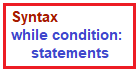The while loop contains an expression/condition. As per the syntax colon (:) is mandatory otherwise it throws syntax error. Condition gives the result as bool type, either True or False. The loop keeps on executing the statements until the condition becomes False.

##### Flowchart for while loop: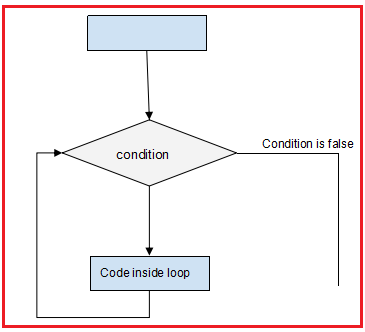##### Parts of while loop in Python:

Initialization: This is the first part of the while loop. Before entering the condition section, some initialization are required

Condition: Once the initializations done, then it will go for condition checking which is the heart of the while loop. The condition is checked and if it returns True, then execution enters the loop for executing the statements inside.

After executing the statements, the execution goes to the increment/decrement section to increment the iterator. Mostly, the condition will be based on this iterator value, which keeps on changing for each iteration. This completes the first iteration.

In the second iteration, if the condition is False, then the execution comes out of the loop else it will proceed as explained in the above point.

Increment/Decrement section: This is the section where the iterator is increased or decreased. Generally, we use arithmetic operators in the loop for this section

##### Let us understand it better with an example

Example: Printing numbers from 1 to 5 by using while loop (demo11.py)

```x=1
while x<=5:
print(x)
x+=1
print("End")```

Output: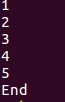In the demo11.py

1. The program is to print the number from 1 to 5
2. Before starting the loop, we have made some assignments( x = 1). This is called the Initialization section.
3. After initialization, we started the while loop with a condition x<=5. This condition returns True until x is less than 5.
4. Inside the loop we are printing the value of x.
5. After printing the x value, we are incrementing it using the operator x+=1. This is called the increment/decrement section.
6. For each iteration the value of x will increase and when the x value reaches 6, then the condition x<=5 returns False. At this iteration, the execution comes out of the loop without executing the statements inside. Hence in the output ‘6’ is not printed.
###### Example: Printing even numbers from 10 to 20 by using while loop (demo12.py)
```x=10
while (x>=10) and (x<=20):
print(x)
x+=2
print("End")```

Output: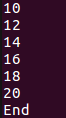##### for loop in python:

Basically, a for loop is used to iterate elements one by one from sequences like string, list, tuple, etc. This loop can be easily understood when compared to while loop. While iterating elements from sequence we can perform operations on every element.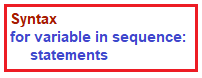###### Example: Printing elements of a list (demo13.py)
```x = [10, 20, 30, "Python"]
for i in x:
print(i)```

Output: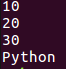###### Example: Printing characters from a string (demo14.py)
```x= "python"
for ch in x:
print(ch)```

Output: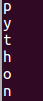###### Example: Printing every item cost by adding gst (demo15.py)
```items_costs = [10, 20, 30,]
gst = 2
for x in items_costs:
print(x+gst)```

Output: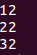###### Example: Printing elements by using range() function and for loop (demo16.py)
```for x in range(1, 5):
print(x)```

Output: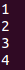###### Example: Printing the sum of elements in a list (demo17.py)
```item_costs = [10, 20, 30]
sum=0
for x in item_costs:
sum=sum + x
print(sum)```

Output: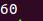##### INFINITE LOOPS in PYTHON

The loop which never ends, or the loop whose condition never gets False is called an infinite loop. This loop never exits. In order to come out of the loop we need to manually do it by command ctrl+c.

###### Example: Infinite loop (demo18.py)
```while True:
print("Hello")```

Output: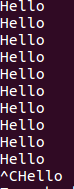##### NESTED LOOPS IN PYTHON

If a loop is written inside another loop then it is called a nested loop. Let’s understand it with an example.

Example: Nested Loops (demo19.py)

```rows = range(1, 5)
for x in rows:
for star in range(1, x+1):
print('* ', end=' ')
print()```

Output: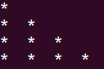##### LOOPS with ELSE block in Python:

In python, loops can also have an else block. These else blocks will get executed irrespective of the loop i.e even if the loop got executed(demo20.py) or not(demo21.py).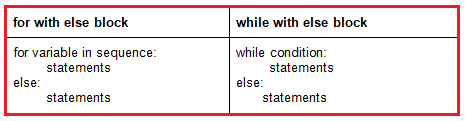###### Example: Loops with else block (demo20.py)
```values = range(5)
for x in values:
print("item is available")
else:
print("sorry boss, item is not available")```

Output: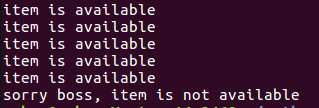###### Example: Loops with else block (demo21.py)
```values = range(5)
for x in values:
print("item is available")
else:
print("sorry boss, item is not available")```

Output: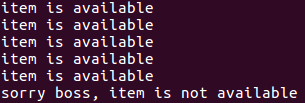Note: These loops with else blocks are best suitable in the scenarios, where we need to search an element in the list. When the element is not present in the list then the else part will be executed saying no such element in the list.

###### Example: Element search in the list (demo22.py)
```group = [1, 2, 3, 4]
search = int(input("Enter the element in search: "))
for element in group:
if search == element:
print("element found in group")
break
else:

Output1: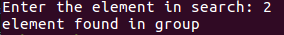Output2: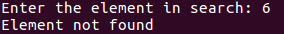##### BREAK statement in Python

The break statement when encountered will terminate the loop and bring the execution out of the loop. Let’s consider the demo22.py, where we are searching for an element in the list. There are four elements in the list and we are searching for element 2. Our search element and the list element gets matched in the second iteration itself. It’s unnecessary to execute the loop for the other two remaining iterations. So, we placed a break statement immediately when the condition is matched in order to skip the remaining iterations.

##### CONTINUE statement in Python

The continue statement when encountered will skip the current iteration and the loop proceeds for the next iteration without executing the statements, which are followed by after continue statement.

Example: Printing the items which are below 500 (demo23.py)

```cart=[10, 20, 500, 700, 50, 60]
for item in cart:
if item>=500:
continue
print("Item: ",item)```

Output: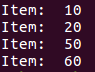##### PASS statement in Python:

The pass statement is used when you have to include some statement syntactically but doesn’t want to perform any operation.

Example: Pass statement (demo24.py)

```num = [10, 20, 30,400, 500, 600]
for i in num:
if i<100:
print("pass statement executed")
pass
else:
print("Give festival coupon for these guys who bought: ",i)```

Output: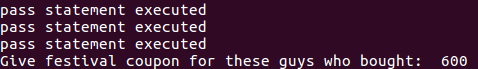##### RETURN statement in Python:

Return statement is one which returns something. This statement is used with functions. We shall deal about this in detail in the functions chapter. For now, just understand that it is one of the statements with which flow can be controlled.